# AMP I.12 – The Common Gate Amplifier

### Introduction

The last of the JFET classical amplifier configuration is the common gate amplifier, analogous to the common base BJT amplifier.  As usual, we will derive the voltage gain, current gain, input resistance, and output resistance of this configuration.

### The Common Gate AmplifierFor the common gate amplifier, the input signal is applied at the source, while the output signal is sampled at the drain.  As you can see, the gate itself is grounded: the gate is “common”.

### Voltage Gain

To derive the voltage gain, let’s draw the small signal equivalent circuit: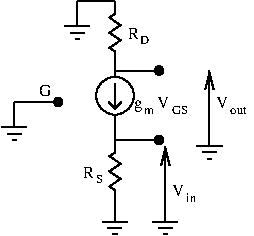As usual, let’s express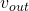andto find the voltage gain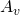:

•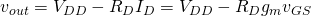•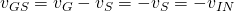, with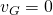because the gate is grounded

Substituting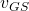in the first equation gives: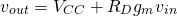We can now calculate our voltage gain: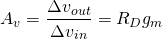If we add a load, this gain becomes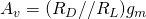### Current Gain

To find the current gain, we need to add a load, and determine the input and output current.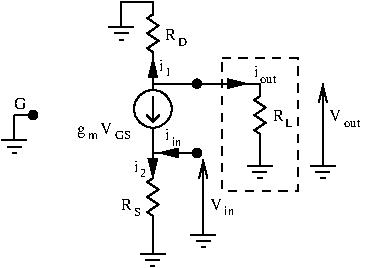Let’s express.  A node equation at the source terminal gives us: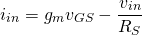Since(gate is grounded), we have: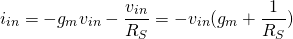Let’s express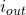next, using ohm’s law.  We already know the output voltagesince it was calculated in the previous section.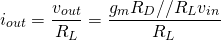We can now derive the current gain: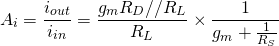By approximating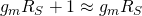, we obtain: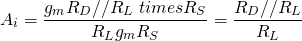As we can see,Our current gain is less than unity.  For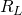relatively low compared to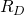, we have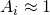.  This is why you will often see people say the common gate amplifier has unity current gain.

### Input Resistance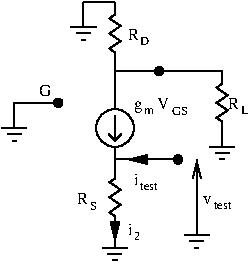To find the input resistance, we apply a test signal at the input and calculate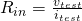:

•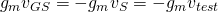•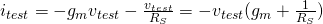, node equation at the source terminal

This gives us: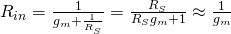.

The input resistance of the common gate amplifier is low.

### Output resistance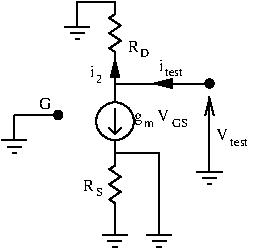To find our output resistance, we remove the load and ground the input.  Then, we apply a test signal at the output and calculate.

Using a node current equation at the drain terminal, we have: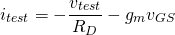However, since the gate and source are grounded (source is grounded because we grounded the input), we have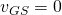.  This gives us: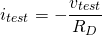And thus, our output resistance is.  This is considered high.

### Conclusion

To recap, the common gate amplifier has:

•, a high voltage gain
•, unity current gain
•, a low input resistance
•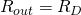, a high output resistance

As expected, the common gate amplifier has very similar properties to the common base amplifier.